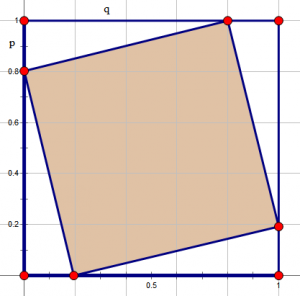Problem A. Square Counting

### Problem

Mr. Panda has recently fallen in love with a new game called Square Off, in which players compete to find as many different squares as possible on an evenly spaced rectangular grid of dots. To find a square, a player must identify four dots that form the vertices of a square. Each side of the square must have the same length, of course, but it does not matter what that length is, and the square does not necessarily need to be aligned with the axes of the grid. The player earns one point for every different square found in this way. Two squares are different if and only if their sets of four dots are different. Mr. Panda has just been given a grid with R rows and C columns of dots. How many different squares can he find in this grid? Since the number might be very large, please output the answer modulo 109 + 7 (1000000007).

### Input

The first line of the input gives the number of test cases, T. T lines follow. Each line has two integers R and C: the number of dots in each row and column of the grid, respectively.

### Output

For each test case, output one line containing Case #x: y, where x is the test case number (starting from 1) and y is the number of different squares can be found in the grid.

### Limits

1 ≤ T ≤ 100.

#### Small dataset

2 ≤ R ≤ 1000. 2 ≤ C ≤ 1000.

#### Large dataset

2 ≤ R ≤ 109. 2 ≤ C ≤ 109.

### Sample

Input

Output

4
2 4
3 4
4 4
1000 500

Case #1: 3
Case #2: 10
Case #3: 20
Case #4: 624937395

The pictures below illustrate the grids from the three sample cases and a valid square in the third sample case.### Problem

Alice likes reading and buys a lot of books. She stores her books in two boxes; each box is labeled with a pattern that matches the titles of all of the books stored in that box. A pattern consists of only uppercase/lowercase English alphabet letters and stars (*). A star can match between zero and four letters. For example, books with the titles GoneGirl and GoneTomorrow can be put in a box with the pattern Gone**, but books with the titles TheGoneGirl, and GoneWithTheWind cannot. Alice is wondering whether there is any book that could be stored in either of the boxes. That is, she wonders if there is a title that matches both boxes’ patterns.

### Input

The first line of the input gives the number of test cases, T. T test cases follow. Each consists of two lines; each line has one string in which each character is either an uppercase/lowercase English letter or *.

### Output

For each test case, output one line containing Case #x: y, where x is the test case number (starting from 1) and y is TRUE if there is a string that matches both patterns, or FALSE if not.

### Limits

1 ≤ T ≤ 50.

#### Small dataset

1 ≤ the length of each pattern ≤ 200. Each pattern contains at most 5 stars.

#### Large dataset

1 ≤ the length of each pattern ≤ 2000.

Input

Output

3
****
It
Shakese
S
speare
Shakese peare
Case #1: TRUE
Case #2: TRUE
Case #3: FALSE

## Problem

“Look at the stars, look how they shine for you.” - Coldplay, “Yellow” In a galaxy far, far away, there are many stars. Each one is a sphere with a certain position (in three-dimensional space) and radius. It is possible for stars to overlap each other. The stars are so incredibly beautiful to you that you want to capture them forever! You would like to build two cubes of the same integer edge length, and place them in space such that for each star, there is at least one cube that _completely_ contains it. (It’s not enough for a star to be completely contained by the union of the two cubes.) A star is completely contained by a cube if no point on the star is outside the cube; a point exactly on a cube face is still considered to be inside the cube. The cubes can be placed anywhere in space, but they must be placed with their edges parallel to the coordinate axes. It is acceptable for the cubes to overlap stars or each other. What is the minimum integer edge length that allows you to achieve this goal?

### Input

The input starts with one line containing exactly one integer T, which is the number of test cases. T test cases follow. Each test case begins with a line containing an integer, N, representing the number of stars. This is followed by N lines. On the ith line, there are 4 space-separated integers, Xi, Yi, Ziand Ri, indicating the (X, Y, Z) coordinates of the center of the ith star, and the radius of the ith star.

### Output

For each test case, output one line containing Case #x: y, where x is the test case number (starting from 1) and y is the minimum cube edge length that solves the problem, as described above.

### Limits

1 ≤ T ≤ 100.
-108 ≤ Xi ≤ 108, for all i.
-108 ≤ Yi ≤ 108, for all i.
-108 ≤ Zi ≤ 108, for all i.
1 ≤ Ri ≤ 108, for all i.

1 ≤ N ≤ 16.

1 ≤ N ≤ 2000.

Input

Output

3
3
1 1 1 1
2 2 2 1
4 4 4 1
3
1 1 1 2
2 3 4 1
5 6 7 1
3
1 1 1 1
1 1 1 1
9 9 9 1

Case #1: 3
Case #2: 5
Case #3: 2